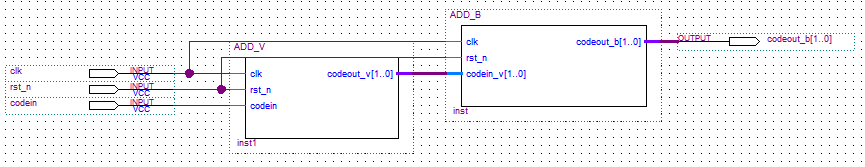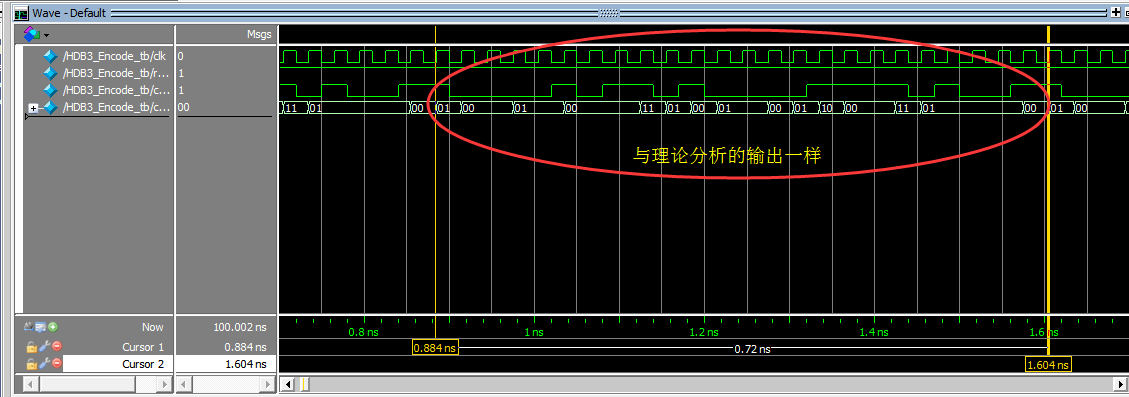# 通信原理---FPGA---HDB3码编码

﻿﻿

https://wenku.baidu.com/view/7cd940274b35eefdc8d3330f.html

https://baike.baidu.com/item/HDB3%E7%A0%81/3815309?fr=aladdin

HDB3码（High Density Bipolar of Order 3），三阶高密度双极性码，它是AMI码的一种改进，目的是克服AMI的缺点，使连续“0”的个数不超过3个。其编码规则如下：

（1） 先检查连“0”个数，若小于等于3，则与AMI码相同；

（2）当连“0”个数大于3时，将每4个连“0”化作一个小节，用“000V”代替，其中V取值+1或-1，V的极性与前一个相邻的非“0”脉冲极性相同（这破坏了极性交替规则，故称V为破坏脉冲）；

（3） 相邻的V极性必须交替，当V满足(2)但不满足(3)时，将“000V”更改为“B00V”，B的极性与后面的V一致，B称调节脉冲，==>即两个相邻V之间的“1”的个数为偶数时V的极性不满足交替，需要替换为“B00V”；为奇数时V的极性能满足交替，不需要替换；

（4）V后面的传号码极性也要交替。

AMI码：  +1  0   0  -1 +1  0   0   0   0  -1   0 +1 -1   0 +1   0   0   0   0 -1 +1 -1 +1   0

01  00  00  01  00  00  00  11  01  00  01  01  00  01  10  00  00  11  01  01  01  01  00

（1）先不考虑极性（+或-），只考虑输出是0、1、B还是V，对上述4个数编码，0--00，1--01，B--10，V--11

（2）加V操作，判断输入的字符是“1”还是“0”，1=>01， 0=>00，若为4个连“0”，前三个输出“00”，第四个输出“11”

always @ ( posedge clk or negedge rst_n )
begin
if( !rst_n )  begin
count0 <= 0;
end
else  begin
if( codein == 1'b1 )  begin  //输入1
codeout_v <= 2'b01;
count0 <= 2'b00;    //出现1后就要重新对0计数
end
else  begin       //输入0
count0 <= count0 + 1'b1; //对0的计数加1
if( count0 == 2'b11 ) begin //0的个数等于3，因为是并行，其实是4个了，只是在下一个时钟上升沿变为4
codeout_v <= 2'b11;  //输出V
count0 <= 2'b00;   //重新对0计数
end
else  begin
codeout_v <= 2'b00;
end
end
end
end

（3）加B操作，判断两个相邻的V之间的非“0”（此时两V之间非0的应该只有1，即“01”）的个数是否为偶数，若为偶数，从后一个V往前数，第三个0变为B；若为奇数，正常输出；

always @ ( posedge clk )
begin
buffer <= codein_v; //移位寄存器延时
buffer <= buffer;
buffer <= buffer;
end

always @ ( posedge clk or negedge rst_n )
begin
if( !rst_n )  begin
count01 <= 0;
first_v <= 0;
end
else  begin
if( codein_v == 2'b11 )  begin  //11-->V
if( first_v == 1'b0 ) begin  //还没出现过V,就不存在两个V之间非0数为偶数的情况，不需要对1计数
first_v <= 1;     //已经出现了V
count01 <= 2'b00;    //出现11后就要重新对01计数
codeout_b <= buffer;  //延时后的输出
end
else  begin
if( count01 == 0 )  begin //1的个数为偶数
codeout_b <= 2'b10;   //B-->10
count01 <= 2'b00;
end
else  begin
codeout_b <= buffer;
count01 <= 2'b00;
end
end
end
else if( codein_v == 2'b01 )  begin  //01--->1
if( first_v == 1'b1 ) begin
count01 <= count01 + 1'b1;
codeout_b <= buffer;
end
end
else begin
codeout_b <= buffer;
end
end
end

（4）极性判断输出，这一步还没做，先写到这，顶层bdf如下（还差个极性转换，有时间再写），Modelsim仿真如下（部分关键代码）initial
begin
#0 clk = 1'b0;
#0 rst_n = 1'b0;
#2 rst_n = 1'b1;
#100000 \$stop;
end

always #15
begin
clk = ~clk;
end

always
begin
#30 codein <= 1'b1;
#30 codein <= 1'b0;
#30 codein <= 1'b0;
#30 codein <= 1'b1;
#30 codein <= 1'b1;
#30 codein <= 1'b0;
#30 codein <= 1'b0;
#30 codein <= 1'b0;
#30 codein <= 1'b0;
#30 codein <= 1'b1;
#30 codein <= 1'b0;
#30 codein <= 1'b1;
#30 codein <= 1'b1;
#30 codein <= 1'b0;
#30 codein <= 1'b1;
#30 codein <= 1'b0;
#30 codein <= 1'b0;
#30 codein <= 1'b0;
#30 codein <= 1'b0;
#30 codein <= 1'b1;
#30 codein <= 1'b1;
#30 codein <= 1'b1;
#30 codein <= 1'b1;
#30 codein <= 1'b0;
end﻿﻿